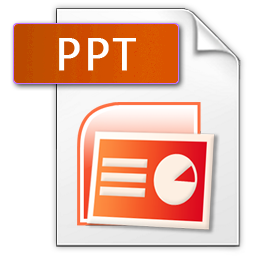# Forces and Newton’s Laws

rating(4 votes, average: 1.50 out of 5)Loading...

This question derives the equation of acceleration of the Atwood Machine. The properties of this interesting machine will then be analyzed., , , , , , ,

rating(1 votes, average: 4.00 out of 5)Loading...

Exploration of free body diagrams, two body acceleration, and Newton’s law through the system of two blocks attached through a pulley and one of them resting on a table., , , , , , ,

rating(2 votes, average: 4.00 out of 5)Loading...

Exploration of free body diagrams, two body acceleration, and newton’s laws through the system of two blocks resting on a pyramid and attached by a pulley., , , , , , , , ,

rating(1 votes, average: 5.00 out of 5)Loading...

Exploration of free body diagrams, two body acceleration, and newton’s laws through the system of two blocks resting on a pyramid and attached by a pulley., , , , , , , , ,

rating(1 votes, average: 5.00 out of 5)Loading...

An introduction to acceleration and newton’s laws using a demonstration of a commuting car., , , , , ,

rating(No Ratings Yet)Loading...

How does a reading on a scale change when on a moving elevator? Scenarios with an elevator moving at different velocities and acceleration will be considered. The concepts learned will then be used to analyze data from a real-life experiment., , , , , , , ,

rating(No Ratings Yet)Loading...

The following set of questions apply Newton’s Second Law to scenarios with multiple blocks held together by the tension force from strings., , , ,

rating(No Ratings Yet)Loading...

What are factors that determine the force of friction? The following set of questions explore the behavior of friction on both moving and stationary objects., , , ,

rating(1 votes, average: 5.00 out of 5)Loading...

Faculty of Education
Scarfe Building
2125 Main Mall,